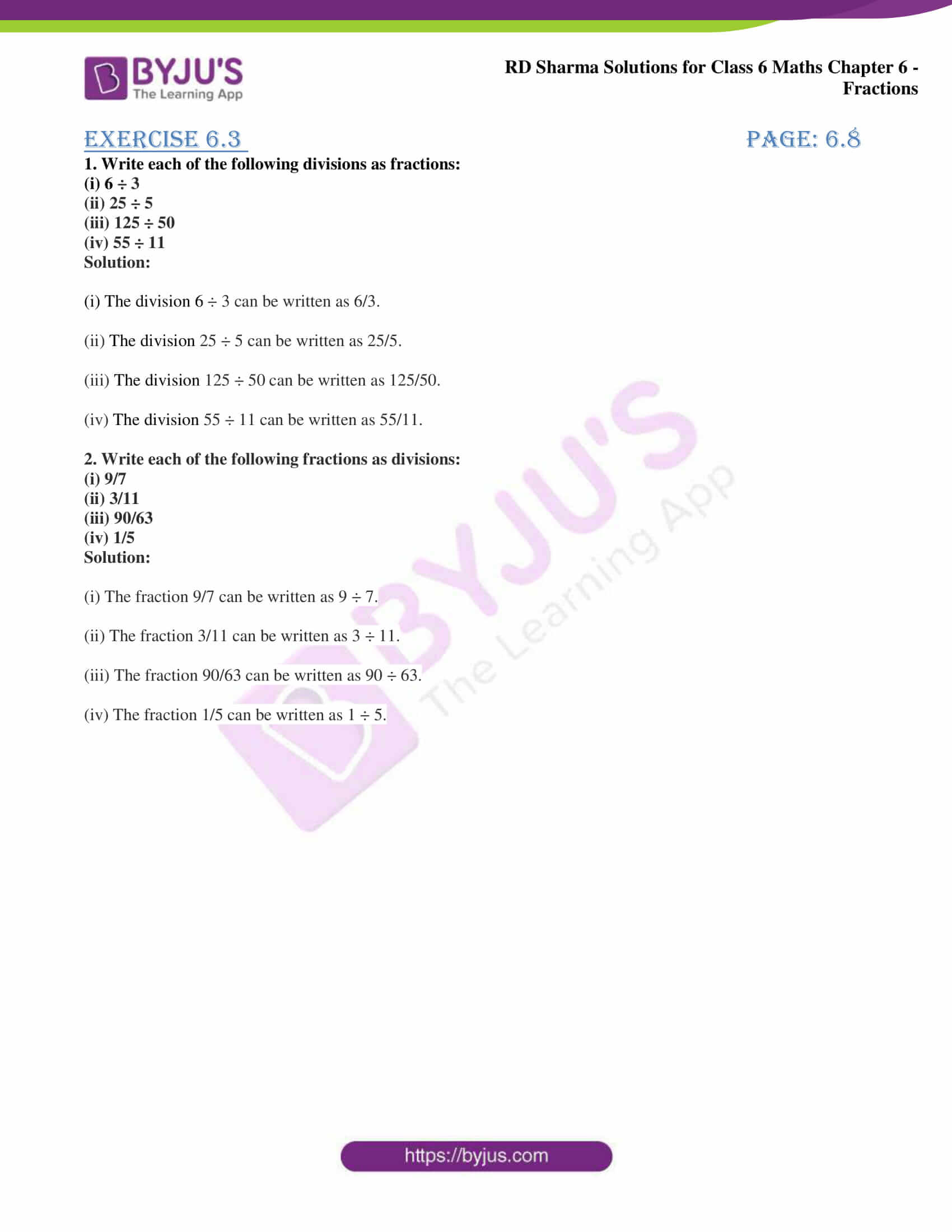# RD Sharma Solutions for Class 6 Maths Chapter 6: Fractions Exercise 6.3

Mathematical concepts of Class 6 are very important as they help in understanding and solving problems of higher classes easily. The students can get their doubts cleared with the help of RD Sharma Solutions Class 6 which are available in PDF format. This exercise explains the methods which are followed in solving the Fractions as division. The students can revise the entire syllabus with the help of PDF and score well in the exam. The dream of students to have a good academic score can be fulfilled with the help of RD Sharma Solutions for Class 6. Students can get the RD Sharma Solutions for Class 6 Maths Chapter 6 Fractions Exercise 6.3 PDF here.

## RD Sharma Solutions for Class 6 Maths Chapter 6: Fractions Exercise 6.3 Download PDF### Access answers to Maths RD Sharma Solutions for Class 6 Chapter 6: Fractions Exercise 6.3

1. Write each of the following divisions as fractions:

(i) 6 ÷ 3

(ii) 25 ÷ 5

(iii) 125 ÷ 50

(iv) 55 ÷ 11

Solution:

(i) The division 6 ÷ 3 can be written as 6/3.

(ii) The division 25 ÷ 5 can be written as 25/5.

(iii) The division 125 ÷ 50 can be written as 125/50.

(iv) The division 55 ÷ 11 can be written as 55/11.

2. Write each of the following fractions as divisions:

(i) 9/7

(ii) 3/11

(iii) 90/63

(iv) 1/5

Solution:

(i) The fraction 9/7 can be written as 9 ÷ 7.

(ii) The fraction 3/11 can be written as 3 ÷ 11.

(iii) The fraction 90/63 can be written as 90 ÷ 63.

(iv) The fraction 1/5 can be written as 1 ÷ 5.